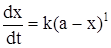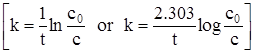# Derive an expression for the rate constant of a first order reaction. Give its units.Posted by

Last updated on August 29th, 2020 at 01:19 am

Let us consider a first order reaction,

A → product

Suppose at the beginning of the reaction, that is, when t=0, the concentration of A is amolLand when t=t, the concentration of A is (a-x)molL.

For a first order reaction, the rate of reaction,where k is the rate constant.Here, c is the constant of integration.

At, t=0, x=0 ; then from equation (i) we have,putting the value of c in equation (i), we get,

ln a – ln(a-x) = ktEquation (ii) is the required expression.

Unit of k:
From equation (ii) we found that the unit of k depends on the unit of t . The unit of k may be s-1, min-1, h-1, day-1 and so on.